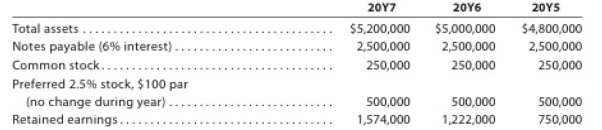Chapter 17, Problem 17.17EX

Chapter
Section
Textbook Problem

Profitability ratiosThe following selected data were taken from the financial statements of Vidahill Inc. for December 31, 20Y7, 20Y6, and 20Y5:The 20Y7 net income was $411,000, and the 20Y6 net income was$462,500. No dividends on common stock were declared between 20Y5 and 20Y7. Preferred dividends were declared and paid in full in 20Y6 and 20Y7.a. Determine the return on total assets, the return tin stockholders’ equity, and the return on common stockholders’ equity for the years 20Y6 and 20Y7. Round percentages to one decimal place.b. What conclusions can be drawn from these data as to the company’s profitability?

a)

To determine

Financial Ratios: Financial ratios are the metrics used to evaluate the liquidity, capabilities, profitability, and overall performance of a company.

To determine: Return on total assets, return on stockholders’ equity and the return on common stockholders’ equity for the years 20Y6 and 20Y7.

Given info: Selected items from the financial statements of V.Inc. for December 31, 20Y7 and 20Y6.

Explanation

Determine return on total assets.

For 20Y7

Rate of return on assets=Netincome + Interest expenseAverage total assets=$411,000+$150,000$5,100,000=11.0% For 20Y6 Rate of return on assets=Netincome + Interest expenseAverage total assets=$462,500+$150,000$4,900,000=12.5%

Return on assets determines the particular company’s overall earning power. It is determined by dividing sum of net income and interest expense and average total assets.

Formula:

Rate of return on assets=Netincome + Interest expenseAverage total assets

Working notes for determining interest expense and average total assets are as follows:

Interest expense for 20Y7

Interest expense for 20Y7 = Notes payable × Interest percentage = $2,500,000 × 6%=$150,000

Interest expense for 20Y6

Interest expense for 20Y6 = Notes payable × Interest percentage = $2,500,000 × 6%=$150,000

Average total assets for 20Y7

Average total assets for 20Y7=Opening assets + Closing assets 2=$5,200,000+$5,000,0002=$5,100,000 Average total assets for 20Y6 Average total assets for 20Y6=Opening assets + Closing assets 2=$5,000,000+$4,800,0002=$4,900,000

Hence, return on assets for 20Y7 and 20Y6 is 11.0% and 12.5%.

Determine return on stockholders’ equity.

Return on stockholders’ equity for 20Y7

Rate of return on stockholders' equity}= Net income Average stockholder’s equity=$411,000$2,148,000=19.1%

Return on stockholders’ equity for 20Y6

Rate of return on stockholders' equity}= Net income Average stockholder’s equity=$462,500$1,736,000=26.6%

Rate of return on stockholders’ equity is used to determine the relationship between the net income and the average common equity that are invested in the company

b)

To determine

To draw: Conclusion about Company’s profitability

Still sussing out bartleby?

Check out a sample textbook solution.

See a sample solution

The Solution to Your Study Problems

Bartleby provides explanations to thousands of textbook problems written by our experts, many with advanced degrees!

Get Started

What is fault tolerance?

Accounting Information Systems

What two kinds of items on a bank reconciliation require journal entries?

College Accounting, Chapters 1-27 (New in Accounting from Heintz and Parry)

Discuss the similarities and differences between the discounted dividend and corporate valuation models.

Fundamentals of Financial Management, Concise Edition (with Thomson ONE - Business School Edition, 1 term (6 months) Printed Access Card) (MindTap Course List)